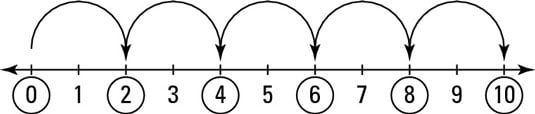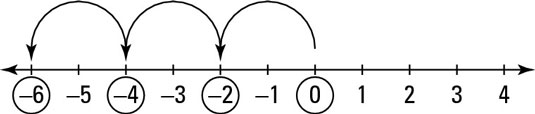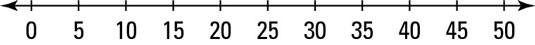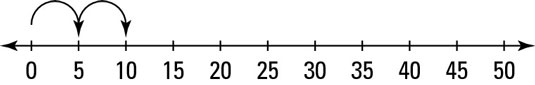##### Basic Math & Pre-Algebra For DummiesSuppose you start at 0 and circle every other number on a number line, as shown in the below figure. As you can see, all the even numbers are now circled. In other words, you’ve circled all the multiples of two. You can now use this number line to multiply any number by two. For example, suppose you want to multiply 5 2. Just start at 0 and jump 5 circled spaces to the right.This number line shows you that 5 2 = 10.

Similarly, to multiply –3 2, start at 0 and jump 3 circled spaces to the left (that is, in the negative direction). The following figure shows you that –3 2 = –6. What’s more, you can now see why multiplying a negative number by a positive number always gives you a negative result.Multiplying on the number line works no matter what number you count off by. For example, the number line in the following figure counts off by 5s.This time, the only numbers marked are the multiples of 5, so you can use this number line to multiply any number by 5. For example, the following figure shows how to multiply 2 5.So 2 5 = 10, the same result as when you multiply 5 2. This result is an example of the commutative property of multiplication — you can reverse the order of any multiplication problem and still get the same answer.